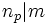# Divisibility condition on Sylow numbers

This article gives the statement, and possibly proof, of a constraint on numerical invariants that can be associated with a finite group

This article states a result of the form that one natural number divides another. Specifically, the (number) of a/an/the (Sylow subgroup) divides the (index) of a/an/the (Sylow subgroup).
View other divisor relations |View congruence conditions

## Statement

Suppose$G$ is a finite group of order:$N = p^km$

where$p$ is prime,$k$ is a nonnegative integer, and$m$ is relatively prime to$p$. Let$n_p$ denote the$p$-Sylow number (?), i.e., the number of$p$-Sylow subgroups of$G$. Then we have:$n_p | m$.